Type
Quiz
Book Title
Fundamentals of Corporate Finance Standard Edition 9th Edition
ISBN 13
978-0073382395

### 978-0073382395 Chapter 19 Questions and Problems

April 3, 2019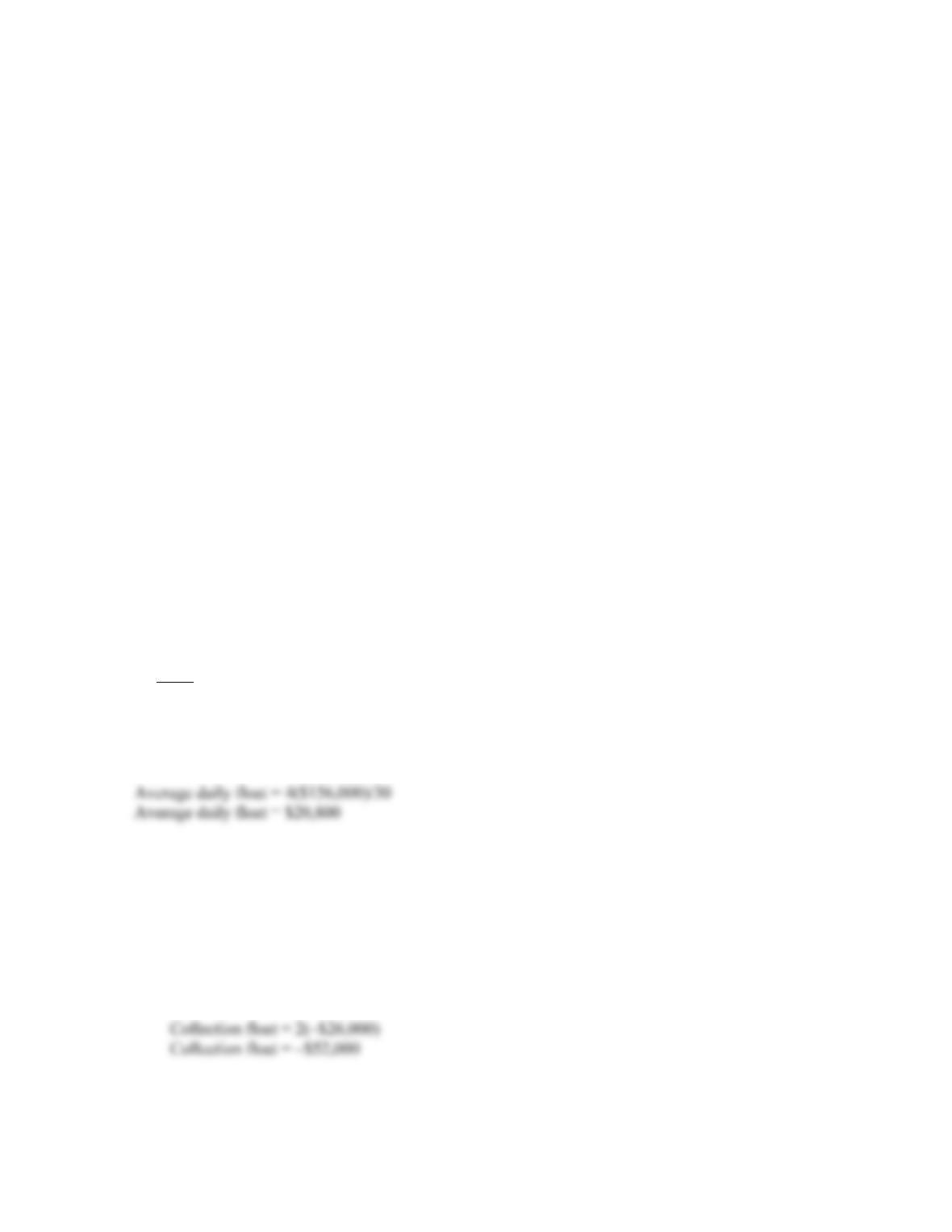CHAPTER 19 B-319
e. The primary disadvantages of RANs is that some possess non-trivial levels of default risk, and
also, corporations are somewhat restricted in the type and amount of these tax-exempts that they
can hold in their portfolios.
f. The primary disadvantage of the repo market is the generally very short maturities available.
10. The concern is that excess cash on hand can lead to poorly thought-out management decisions. The
thought is that keeping cash levels relatively low forces management to pay careful attention to cash
flow and capital spending.
11. A potential advantage is that the quicker payment often means a better price. The disadvantage is that
doing so increases the firm’s cash cycle.
12. This is really a capital structure decision. If the firm has an optimal capital structure, paying off debt
moves it to an under-leveraged position. However, a combination of debt reduction and stock buy-backs
could be structured to leave capital structure unchanged.
13. It is unethical because you have essentially tricked the grocery store into making you an interest-free
loan, and the grocery store is harmed because it could have earned interest on the money instead of
loaning it to you.
Solutions to Questions and Problems
NOTE: All end of chapter problems were solved using a spreadsheet. Many problems require multiple steps.
Due to space and readability constraints, when these intermediate steps are included in this solutions
manual, rounding may appear to have occurred. However, the final answer for each problem is found
without rounding during any step in the problem.
Basic
1. The average daily float is the average amount of checks received per day times the average number of
days delay, divided by the number of days in a month. Assuming 30 days in a month, the average daily
float is:
2. a. The disbursement float is the average monthly checks written times the average number of days for
the checks to clear, so:
Disbursement float = 4(\$14,000)
Disbursement float = \$56,000
The collection float is the average monthly checks received times the average number of days for
the checks to clear, so: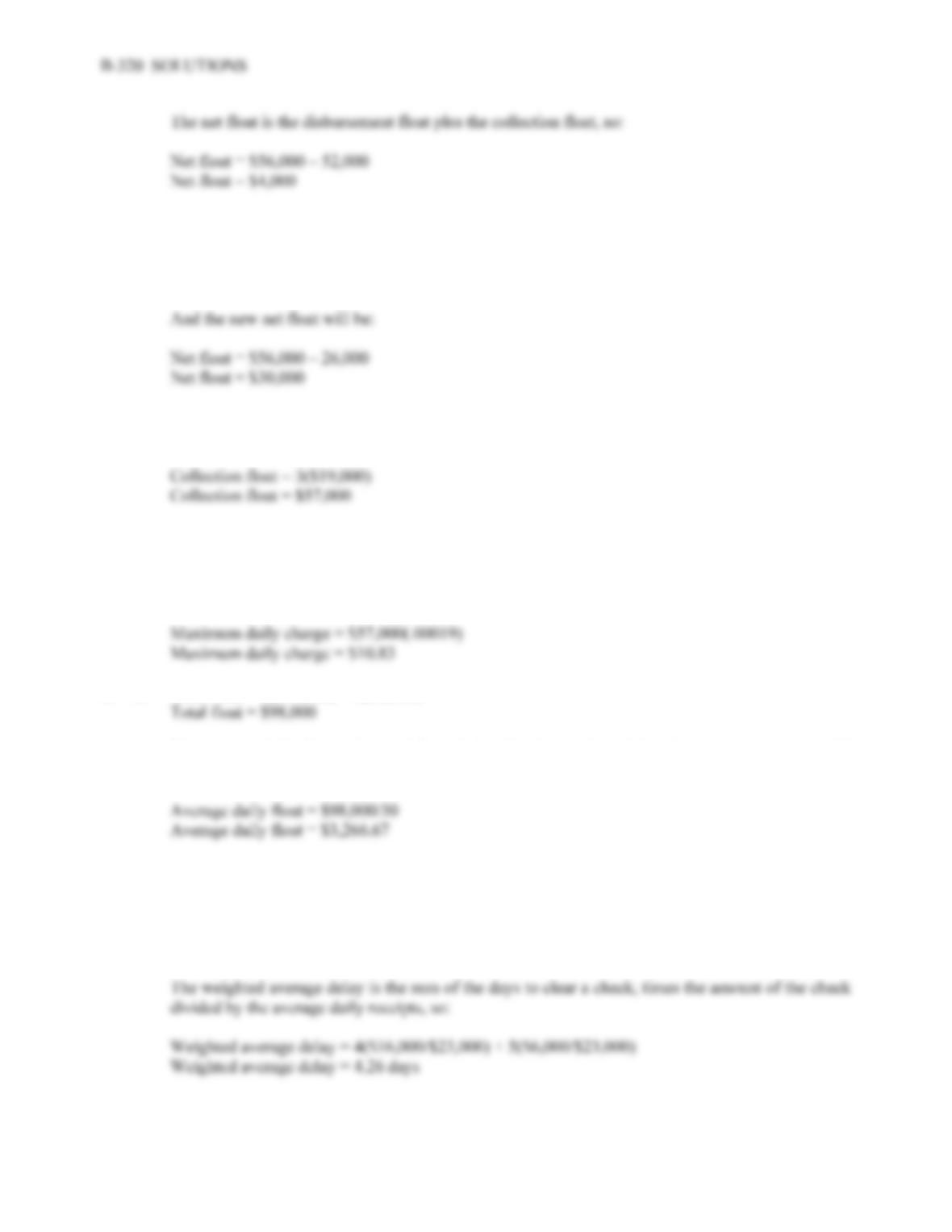b. The new collection float will be:
Collection float = 1(–\$26,000)
Collection float = –\$26,000
3. a. The collection float is the average daily checks received times the average number of days for the
checks to clear, so:
b. The firm should pay no more than the amount of the float, or \$57,000, to eliminate the float.
c. The maximum daily charge the firm should be willing to pay is the collection float times the daily
interest rate, so:
4. a. Total float = 4(\$17,000) + 5(\$6,000)
b. The average daily float is the total float divided by the number of days in a month. Assuming 30
days in a month, the average daily float is:
c. The average daily receipts are the average daily checks received divided by the number of days in
a month. Assuming a 30 day month:
Average daily receipts = (\$17,000 + 6,000)/30
Average daily receipts = \$766.67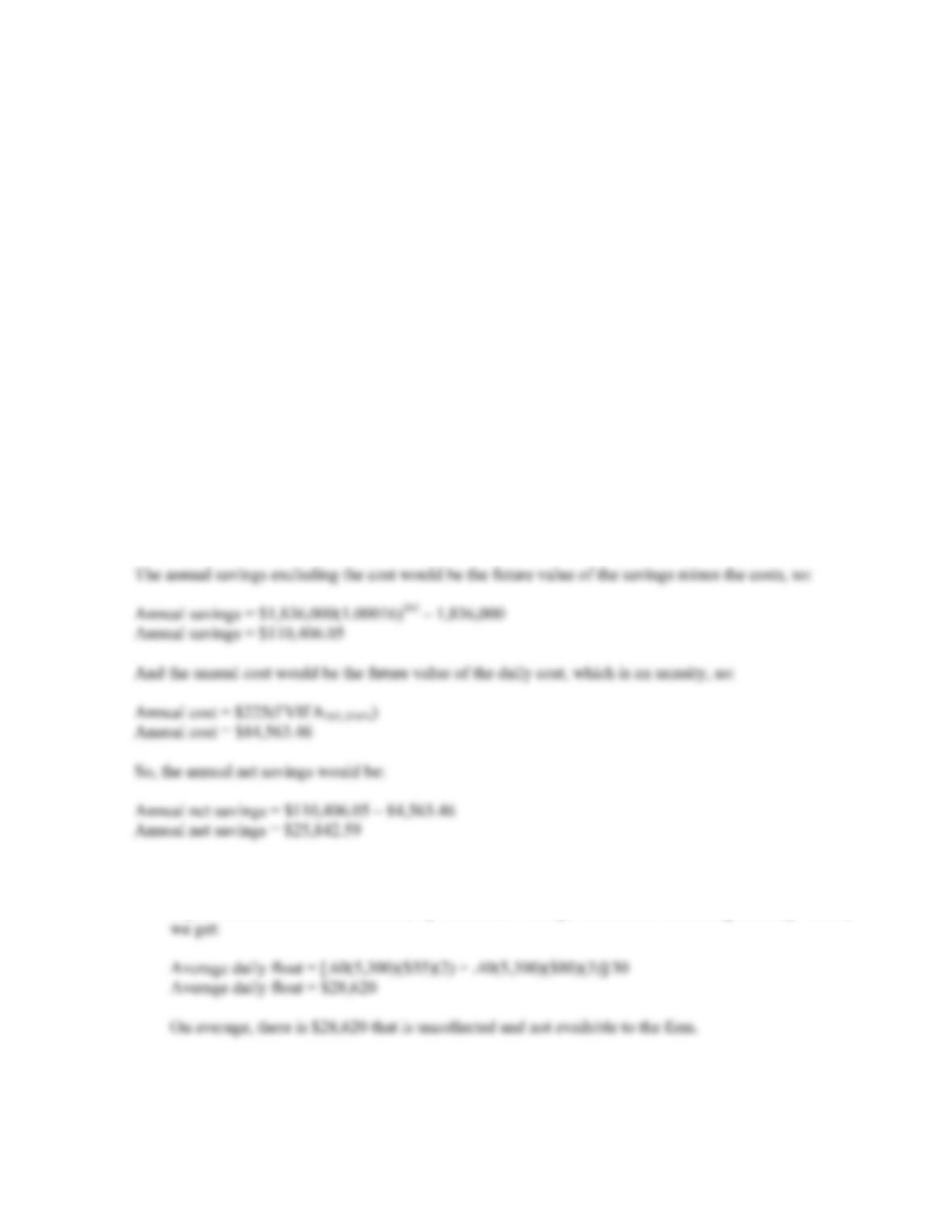CHAPTER 19 B-321
5. The average daily collections are the number of checks received times the average value of a check, so:
Average daily collections = \$108(8,500)
Average daily collections = \$918,000
The present value of the lockbox service is the average daily receipts times the number of days the
collection is reduced, so:
PV = (2 day reduction)(\$918,000)
PV = \$1,836,000
The daily cost is a perpetuity. The present value of the cost is the daily cost divided by the daily interest
rate. So:
PV of cost = \$225/.00016
PV of cost = \$1,406,250
The firm should take the lockbox service. The NPV of the lockbox is the cost plus the present value of
the reduction in collection time, so:
NPV = –\$1,406,250 + 1,836,000
NPV = \$429,750
6. a. The average daily float is the sum of the percentage each check amount is of the total checks
received times the number of checks received times the amount of the check times the number of
days until the check clears, divided by the number of days in a month. Assuming a 30 day month,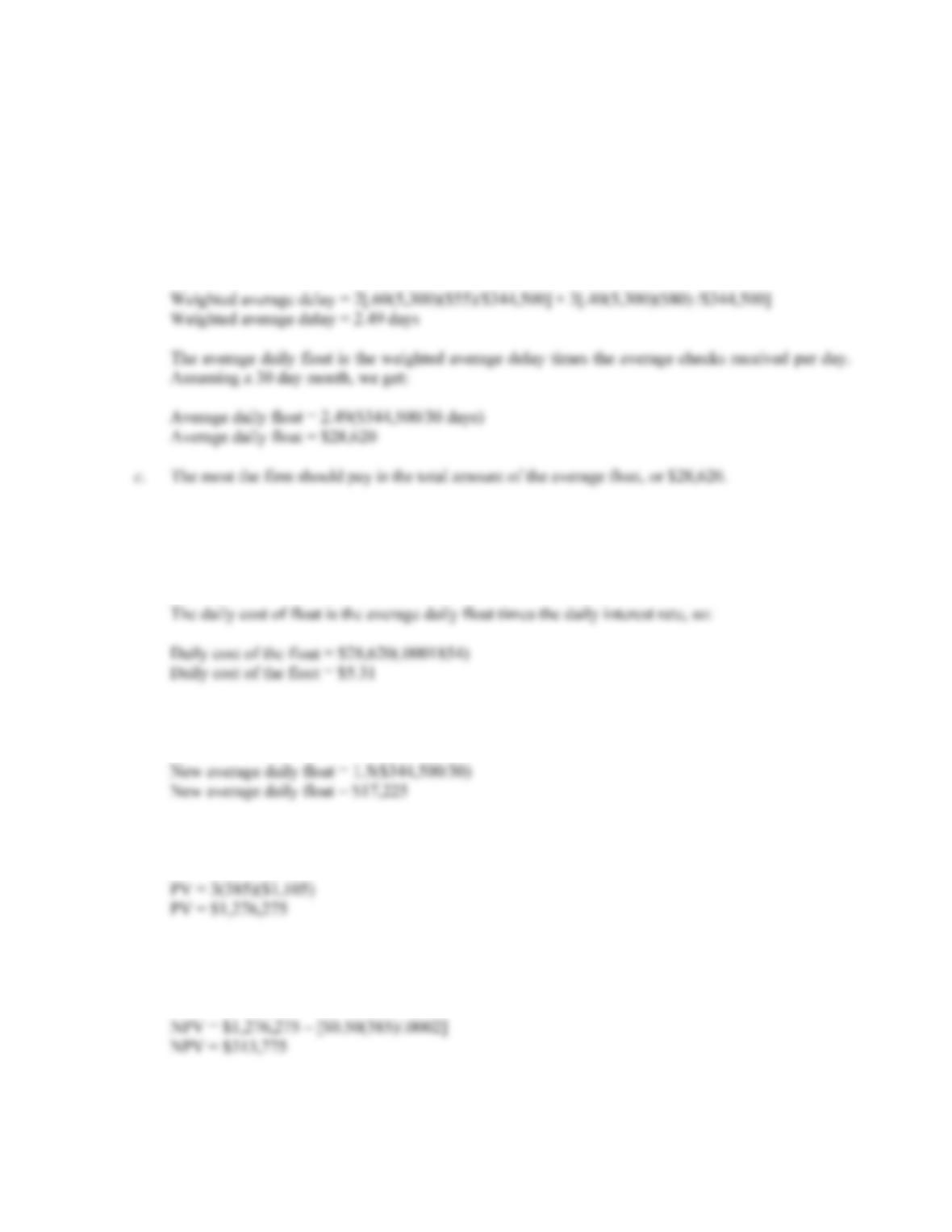B-322 SOLUTIONS
b. The total collections are the sum of the percentage of each check amount received times the total
checks received times the amount of the check, so:
Total collections = .60(5,300)(\$55) + .40(5,300)(\$80)
Total collections = \$344,500
The weighted average delay is the sum of the average number of days a check of a specific amount
is delayed, times the percentage that check amount makes up of the total checks received, so:
d. The average daily interest rate is:
1.07 = (1 + R)365
R = .01854% per day
e. The most the firm should pay is still the average daily float. Under the reduced collection time
assumption, we get:
7. a. The present value of adopting the system is the number of days collections are reduced times the
average daily collections, so:
b. The NPV of adopting the system is the present value of the savings minus the cost of adopting the
system. The cost of adopting the system is the present value of the fee per transaction times the
number of transactions. This is a perpetuity, so: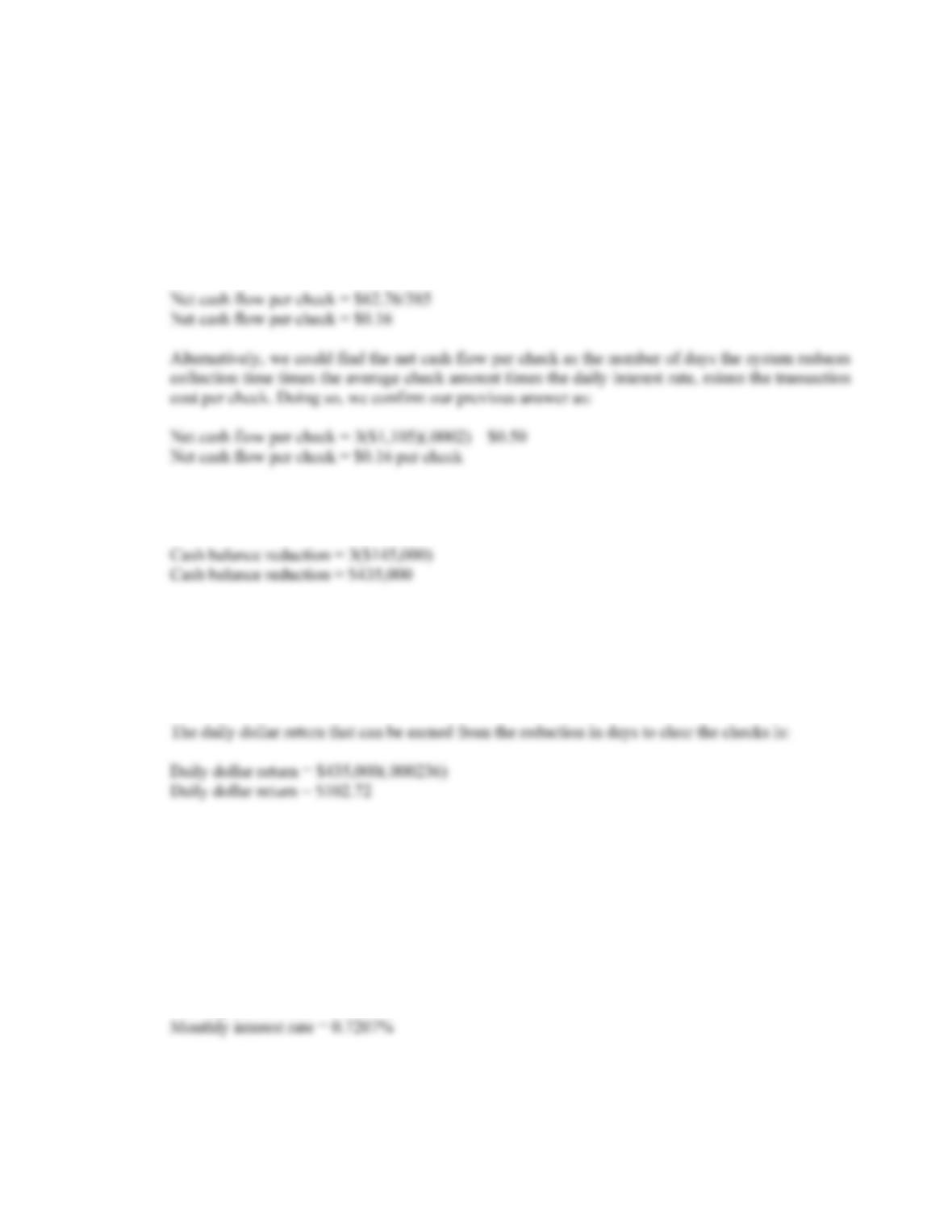CHAPTER 19 B-323
c. The net cash flows is the present value of the average daily collections times the daily interest rate,
minus the transaction cost per day, so:
Net cash flow per day = \$1,276,275(.0002) – \$0.50(385)
Net cash flow per day = \$62.76
The net cash flow per check is the net cash flow per day divided by the number of checks received
per day, or:
8. a. The reduction in cash balance from adopting the lockbox is the number of days the system reduces
collection time times the average daily collections, so:
b. The dollar return that can be earned is the average daily interest rate times the cash balance
reduction. The average daily interest rate is:
Average daily rate = 1.091/365 – 1
Average daily rate = .0236% per day
c. If the company takes the lockbox, it will receive three payments early, with the first payment
occurring today. We can use the daily interest rate from part b, so the savings are:
Savings = \$145,000 + \$145,000(PVIFA.0236%,2)
Savings = \$434,897.32
If the lockbox payments occur at the end of the month, we need the effective monthly interest rate,
which is:
Monthly interest rate = 1.091/12 – 1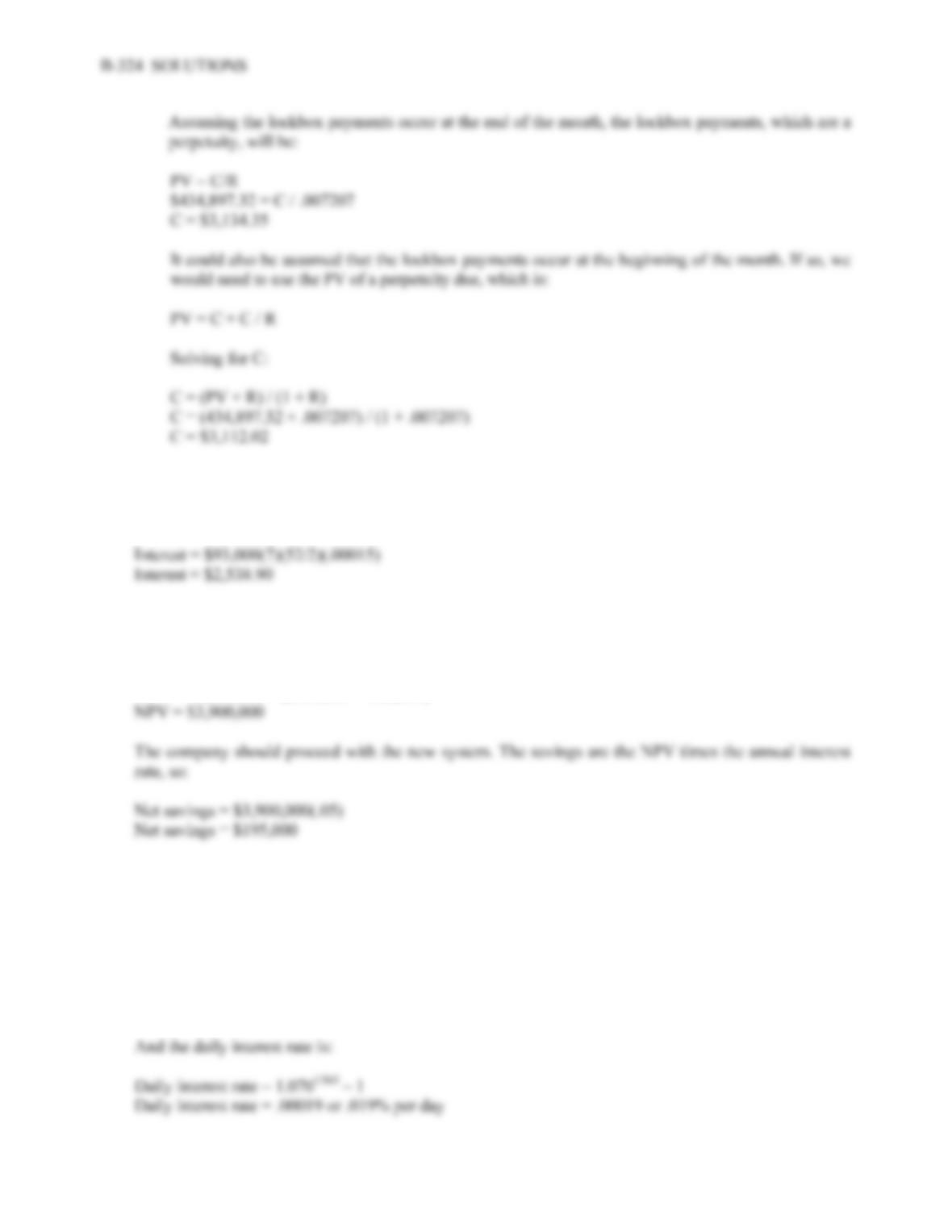9. The interest that the company could earn will be the amount of the checks times the number of days it
will delay payment times the number of weeks that checks will be disbursed times the daily interest rate,
so:
10. The benefit of the new arrangement is the \$4 million in accelerated collections since the new system
will speed up collections by one day. The cost is the new compensating balance, but the company will
recover the existing compensating balance, so:
NPV = \$4,000,000 – (\$400,000 – 500,000)
Intermediate
11. To find the NPV of taking the lockbox, we first need to calculate the present value of the savings. The
present value of the savings will be the reduction in collection time times the average daily collections,
so:
PV = 2(750)(\$980)
PV = \$1,470,000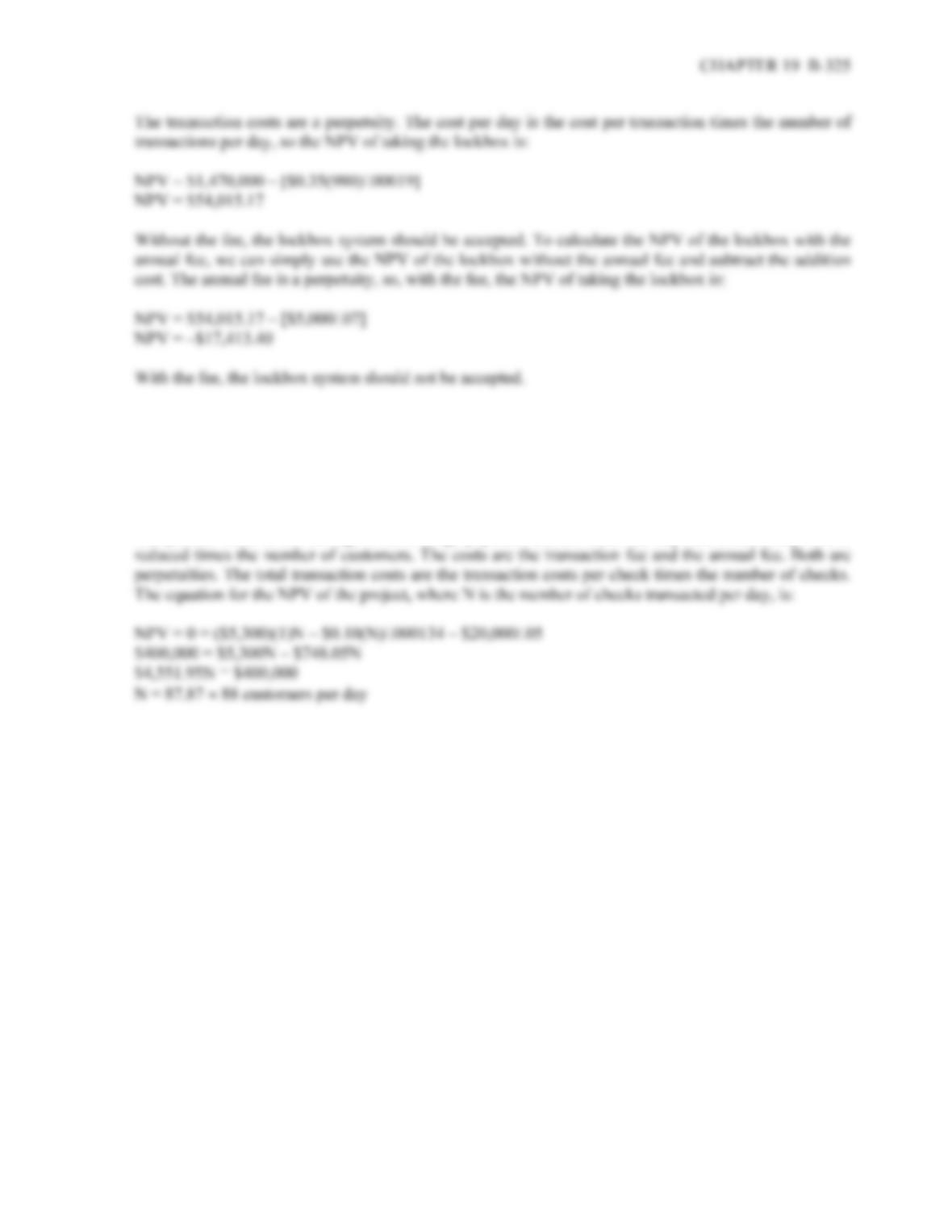12. To find the minimum number of payments per day needed to make the lockbox system feasible is the
number of checks that makes the NPV of the decision equal to zero. The average daily interest rate is:
Daily interest rate = 1.051/365 – 1
Daily interest rate = .0134% per day
The present value of the savings is the average payment amount times the days the collection period is